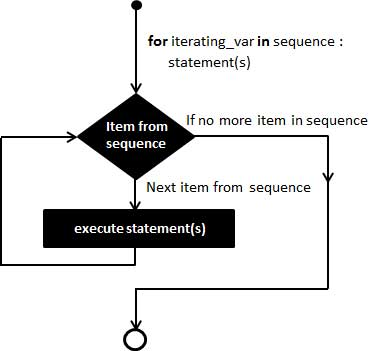# 循环类型

PHP 中的循环语句用于执行指定次数相同的代码块。PHP 支持以下四种循环类型：

• for – 循环执行代码块指定的次数
• while – 只要指定的条件为真，则循环执行代码块
• do…while – 循环执行代码块，然后在指定的条件为真时重复这个循环
• foreach – 根据数组中每个元素来循环代码块

## for 语句## 语法

``````    for (initialization; condition; increment)
{
code to be executed;
}``````

## 例子

``````    <html>
<body>
<?php
\$a = 0;
\$b = 0;

for( \$i=0; \$i<5; \$i++ )
{
\$a += 10;
\$b += 5;
}
echo ("At the end of the loop a=\$a and b=\$b" );
?>
</body>
</html>``````

``    At the end of the loop a=50 and b=25``

## 语法

``````    while (condition)
{
code to be executed;
}``````

## 例子

``````    <html>
<body>
<?php
\$i = 0;
\$num = 50;

while( \$i < 10)
{
\$num--;
\$i++;
}
echo ("Loop stopped at i = \$i and num = \$num" );
?>
</body>
</html>``````

``    Loop stopped at i = 10 and num = 40``

## do_while 循环语句

do…while 语句会至少执行一次代码 – 然后，只要条件为真，就会重复进行循环。

### 语法

``````    do
{
code to be executed;
}
while (condition); ``````

### 例子

``````    <html>
<body>
<?php
\$i = 0;
\$num = 0;
do
{
\$i++;
}while( \$i < 10 );
echo ("Loop stopped at i = \$i" );
?>
</body>
</html>``````

``    Loop stopped at i = 10``

## foreach 语句

foreach 语句用于循环遍历数组。每进行一次循环，当前数组元素的值就会被赋值给 value 变量，数组指针会逐一地移动以此类推。

### 语法

``````    foreach (array as value)
{
code to be executed;
}``````

### 例子

``````    <html>
<body>
<?php
\$array = array( 1, 2, 3, 4, 5);
foreach( \$array as \$value )
{
echo "Value is \$value <br />";
}
?>
</body>
</html>``````

``````    Value is 1
Value is 2
Value is 3
Value is 4
Value is 5``````

## Break语句

PHP 关键字 break 被用来结束当前循环。

break 语句坐落在语句块。如果给你完全控制，每当你想退出本次循环。走出一个循环语句立即将被执行。

### 例子

``````    <html>
<body>

<?php
\$i = 0;

while( \$i < 10)
{
\$i++;
if( \$i == 3 )break;
}
echo ("Loop stopped at i = \$i" );
?>
</body>
</html>``````

``    Loop stopped at i = 3``

## Continue语句

PHP continue 关键字在循环结构中用来跳过本次循环但它不会终止循环。

continue 语句像 break 语句一样坐落在包含循环执行的代码块中。通过遇到的 continue 关键字，跳过本次循环剩下的循环代码，执行下次循环。

### 例子

``````    <html>
<body>
<?php
\$array = array( 1, 2, 3, 4, 5);
foreach( \$array as \$value )
{
if( \$value == 3 )continue;
echo "Value is \$value <br />";
}
?>
</body>
</html>``````

``````    Value is 1
Value is 2
Value is 4
Value is 5``````

Posted in PHP教程